## Artigo

•Citado por SciELO
•Acessos

•Similares em SciELO

## versão impressa ISSN 0035-001X

### Rev. mex. fis. vol.66 no.1 México Jan./Fev. 2020  Epub 27-Nov-2020

#### https://doi.org/10.31349/revmexfis.66.69

Research

Fluid Dynamics

Experimental and numerical study of submerged jets from pipes of different wall thicknesses for Re < 1

aInstituto Politécnico Nacional, SEPI ESIME Zacatenco, Av. Miguel Othon de Mendizabal SN, La Escalera, México City, México. e-mail: foxandkirvi@hotmail.com; casillas.juan@hotmail.com; josealfredo1474@yahoo.com

bDepartment of Energy, CIEMAT, Avda. Complutense 22, Madrid 28040, Spain e-mail: vadim.k@ciemat.es

cETS Ingeniería Aeronáutica y del Espacio, UPM, Plaza del Cardenal Cisneros 3, 28040 Madrid, Spain. and Instituto Politécnico Nacional, SEPI ESIME Azcapotzalco, Av. de las Granjas 682, Col. Santa Catarina, Azcapotzalco 02250, México City, México. e-mail: amedinao@ipn.mx

Abstract

In this work, the experimental and numerical results from the study of the effects caused in a submerged jet flow by the change in the wall thickness of the circular tube from which said flow originates are presented. For small values of the Reynolds number Re (Re ≈ 0:11), four cases, regarding the ratio of pipe wall thickness to inner radius, are considered: (I) pipe thickness is a fourth of the radius, (II) pipe thickness is half the radius, (III) pipe thickness is equal to the radius and (IV) pipe thickness is three times the radius. The Particle Image Velocimetry (PIV) technique was used to experimentally obtain the velocity and streamlines distributions. A numerical code based on the finite difference method was developed to solve the motion governing equations, and the numerical results were compared with the values obtained experimentally.

Keywords: Low-Reynolds-number (creeping) flows; computational methods in fluid dynamics; velocity measurements; PIV

PACS: 47.15.G-; 47.11.-j; 47.80.Cb

1.Introduction

The submerged jet flow has been a widely studied subject due to its frequent occurrence in nature and its multiple applications in engineering. Based on the Reynolds number (Re) value, two main types of submerged jet flows can be defined, namely, laminar and turbulent. Some examples of turbulent jets (Re> 3000) can be found in the breathing process in the human nasal passages (Doorly et al.1), among others. Similarly, laminar jets (Re< 3000) can be found in the propulsion drive of some animals such as jellyfish (Vogel 2). The fundamental interest of this work is the study of the dynamics of submerged jet flows for small values of the Reynolds number (Re< 1) and the changes in the structure of the flow that take place when it leaves the tube of a given wall thickness.

To our knowledge, the first exact solutions for submerged jet flows induced by a point source of momentum within an infinite medium were proposed by Landau 3, Squire4 and Yatseyev 5.

Landau3 made the following assumptions to solve this problem analytically: the jet stream is produced by a point source of momentum, which implies that there is no mass transfer; the flow is in a steady-state; the fluid that occupies the medium in which the jet discharges and fluid injected by the jet are the same; the fluid is highly viscous; finally, the external forces that could act on the flow are not taken into account. This solution has two limiting cases, -first, when the value of Re is very low (weak flow) and -second, when the value of Re is very high (strong flow), this idealized flow was proposed as an example of an academic problem with exact solution, but it is physically unrealistic.

Regarding practical cases, some of the first experimental studies on submerged jet streams that originate from a pipe were carried out by Oberbeck , in his study, a solution of water and ink was injected vertically with ascending direction, first continuously and then intermittently, the evolution in time of the jet was sketched, the vortex ring that is generated during the very early stages of the injection process is described and changes on the structure of the jet when interacting with different obstacles, such as a sphere, a wall normal to the flow and a wall parallel to the flow, were discussed.

Similarly, Reynolds 7 made observations on submerged jet streams, this time, he also injected fluid vertically, but along the downward direction and described how the experimental conditions made it difficult to obtain steady flows for Reynolds numbers between 10 and 30.

Abramovich and Solan8 conducted experiments in the range from 80 <Re< 500, finding that the forward speed of the front is proportional to half the speed of the fluid element on the longitudinal axis of the steady jet, this velocity was measured throughout an axial distance of 40 diameters from the pipes’ outlet .

Andrade and Tsien 9 captured long exposure photographs of a horizontally injected jet of a fluid containing suspended particles, obtaining the velocity profile in the center-line of the jet.

Other authors have conducted experiments on submerged jet flows that originate in a hole in a wall instead of a tube. McNaughton and Sinclair 10, agree with Reynolds7 that the action of dyeing the fluid sensitively modifies its density, which becomes evident for small Re values (Re< 100), consequently, the fluid modifies the behavior of the jet, because the flotation and the inertial forces are of the same order of magnitude. Schneider11 and Zauner12 found two different behaviors of the jet, first, when Re> 30, the flow is perpendicular to the walls due to the viscosity, and second, when Re< 30 two toroidal eddies are formed due to the influence of the walls. Schneider13 presents a theoretical prediction that describes the flow with more accuracy, for these cases, compared to the Squire4 solution.

In more recent works on the submerged jet problem, Lemanov et al.14 studied the transition distance of the submerged jets from laminar to turbulent for 100 >Re> 600. In their experiments, they observed that the width of the jet is the same size as that of the diameter of the injector.

Hsu et al. 15 carried out experiments of a submerged jet that ensues from a pipe for Re= 39.4 and 78.8, they find that the jet flow goes in the opposite direction to the injection soon after leaving the source, this is attributed to the difference of densities between the injected flow and the surrounding environment. Lee etal. developed an analytical solution for fully developed laminar jets, by which the maximum velocity in the center of the injector can be calculated.

The aforementioned authors studied the influence on the flow structure due to the change in the value of Re number for injectors of fixed shape, nevertheless, in the present work, the effects on the flow structure due to the change in wall thickness are studied, for this purpose, the division of this work is as follows: the physical problem is stated in Sec. 2, the equations to be solved are given, as well as the conditions to which they are subject, the method used in their solution is described, likewise, the experimental configuration is described along with the procedure followed during measurements. Section 3 presents the most significant results obtained from the numerical simulations and the experimental realizations in the form of contour graphs and velocity components distribution plots, as well as the stream function value distribution; finally, in the last section, the main results obtained in this study are discussed.

2.Numerical solution and experimental study

2.1.Problem statement

In order to determine the submerged jet flow structure, the equations of continuity and Navier-Stokes (N. S.), which express the conservation of mass and momentum, respectively, were stated in cylindrical coordinates and were solved taking the following considerations: the flow is assumed to be axisymmetric; the jet emerges from a submerged circular pipe, which discharges the same fluid found in the quiescent surrounding medium; the fluid is considered to be Newtonian, incompressible, with constant viscosity and density; the flow is assumed to have reached a steady-state; the gravity force, as well as all external forces, are absent; (see the sketch in Fig. 1) leaving: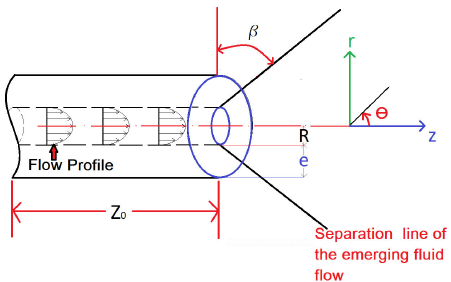FIGURE 1 Schematic representation of the pipe outlet, spacial coordinates and geometrical dimensions.

Continuity equation in cylindrical coordinates

1r(rvr)r+z(ρvz)=0, (1)

and the respective N.S. equation in cylindrical coordinates are coordinate r:

vrvrr+vzvrz=-1ρpr+νu(Δvr-vrr2), (2)

coordinate z:

vrvzr+vzvzz=-1ρpr+νΔvz, (3)

where

Δf=1rr(rfr)+2fz2. (4)

From Eqs. (1)-(3) the vorticity-stream function formulation in cylindrical coordinates is obtained

1rψzςr-1rψrςz-1rψzςr=1Re2ςz2+2ςr2+1rςr-1r2ς (5)

where

ς=(1r2ψr-1r2ψr2-1r2ψz2), (6)

And

vr=1rψz,vz=-1rψr. (7)

The equations were dimensionalized using the inner radius of the tube R and the value of the mean velocity v mean =Q/πR 2 , where the Reynolds number based on R is Re=ρv mean R/μ. Equations (5) and (6) are the same as those used by Kanda and Shimomukai 17, in the numerical treatment of the entrance flow problem in a pipe.

2.2.Numerical method

The vorticity-stream function formulation, which consists of a set of coupled equations, (namely, the vorticity transport Eq. (5) and the Poisson equation for vorticity (6)), was numerically solved for ψ and ς through the implicit successive over-relaxation Gauss-Seidel method, similar to that used by Kanda and Shimomukai17 .

The boundary conditions for ψ and ς at the inlet are set to match a fully developed Poseuille flow

ψ=r2-r42,ς=4r. (8)

The boundary conditions for the vorticity and the stream function representing the solid walls satisfy the non-slip and non-penetration conditions, respectively

ψwall0.5. (9)

At the walls, the vorticity boundary conditions are set to be: for all solid vertical (longitudinal) boundaries

ς=-1r2ψz2, (10)

for all solid horizontal (transversal) boundaries

ς=-1r2ψr-1r2ψr2, (11)

for the center line, the boundary conditions are set to be

ψ=0,ς=0. (12)

Finally, the lineal extrapolation function is applied at the flow outlet

2ψz2=0,2ςz2=0. (13)

To apply the numerical method, the central nodes were discretized with centered second order finite differences. Depending on each boundary, either a forward or backward second-order discretization was implemented. At every iteration, the residues for the vorticity and stream function were computed. An iteration convergence criterion was implemented based on the residual value of the stream function, in which the code stops iterating when the absolute value of the difference of the stream function between the last two iterations is less than or equal to 1×10−8. The code was executed for the following four mesh sizes, 100×100, 200×200, 400×400 and 1000×1000, having found that between the last two meshes there is no significant change in the stream function distribution, the 400×400 mesh was chosen to save computing resources.

2.3.Experimental set up

In order to carry out the experimental study and to analyze the submerged jet flow structure, a prismatic tank with transparent 6 mm thick acrylic walls was built with internal dimensions 11.2×11.8×60 mm (see Fig. 2), these dimensions were chosen to minimize the effects caused by the proximity of the walls to the jet flow, which can be avoided with a separation of at least 6 times the radius according to López-Villa et al., , with said separation being 17 times the inner radius for the present study. At the base of the container, an 8 mm circular hole was drilled allowing the injector pipe to pass through, to determine the injection flow rate, the left wall of the channel was marked with multiple horizontal lines with a separation of 2 mm. Four aluminum tubes were machined, all of them with an inner diameter D in = 6.35 mm ±0.02 mm (R= 3.175 mm) and outer diameters D out = 8 mm ±0.02 mm, 9.52 mm ±0.02 mm, 12.69 mm ±0.0.02 mm and 24.5 mm ±0.0.02 mm, and 100 mm length to achieve fully developed flow inside the pipe and produce a parabolic velocity profile (Poisseuille flow), all four pipes at the opposite end have an outer diameter of 8 mm so they can be coupled at the reservoir base.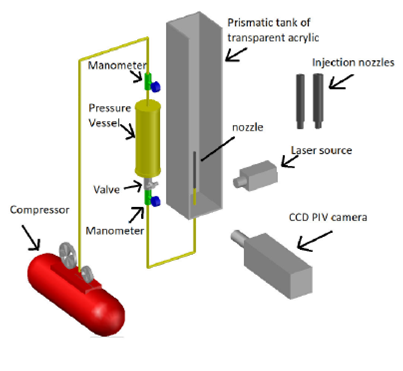FIGURE 2 Schematic representation of the experimental set up.

The working fluid consisted of U.S.P. glycerin, with dissolved 20 μm polyamide, thus allowing the use of the PIV technique. The fluid was injected using a pressurized vessel, which has a capacity of 500 ml. Injection pressure for all cases was set at about 0.6 kg/cm2, to obtain Re≈ 0.11 values, the pressure was measured with a Metron brand pressure gauge with a capacity of 1 kg/cm2, with an accuracy of ±0.5%. The vessel was pressurized with a “maintenance free” Craftsman brand compressor, which was equipped with a moisture filter, and has a 33 Gal storage capacity. The velocity fields were video recorded with a Dantec Dynamics PIV system, and the image sets were processed using the DANTEC Dynamic Studio software. The video camera is set normal to the laser sheet, in turn, the laser sheet is placed at the middle plane of the test section, and it crosses the pipe centerline.

2.4.Experimental procedure

The experimental method used for video recording the flow structure was as follows: the pressure vessel was filled with 0.5 liters of glycerin with dissolved polyamide as tracer particles, pressure in the vessel is raised with compressed air injected at the air chamber of a pressured vessel which was supplied by the compressor until a pressure of 0.6 kg/cm2 was reached, the valve was fully opened and the pressure drop was measured, simultaneously PIV recordings were initiated, thus capturing the transient state of the submerged jet flow. After the first 10 minutes of injection, changes in the flow structure were no longer perceived, and it was considered that a steady-state was achieved.

To compute the flow rate, the time that the free surface took to reach each one of the evenly spaced markings (2 mm) was measured, along with the time it took to empty the 500 ml from the pressurized vessel.

Properties of glycerin such as density, dynamic and kinematic viscosity, as a function of temperature, were interpolated from tables given elsewhere . To reduce the amount of water absorbed by the glycerin from the environment, at the end of each experiment, the glycerin was heated up to 150C, using a “Felisa” brand model FE− 311 thermoshaker. The experiment was set to rest for up to four hours, so the fluid would be still before the jet was injected in the following experiment.

3.Experimental and numerical results

The main objective of the series of experiments described here is to register the shape of a submerged jet flow for a small Reynolds number (Re= 0.11) and to describe how said shape changes with increasing the wall thickness of the injector. The above is achieved by using four nozzles with the same internal diameter but different wall thicknesses e (corresponding to e=R/4, R/2, R and 3R).

Three important changes in the shape of the jet, which can be observed with the naked eye, are presented in Fig. 3. First, the change in the exit angle β of the flow at the injector mouth, this angle decreases with the thickness of the injector wall (see Fig. 4); second, as the flow moves away from the injector along the line of symmetry, the jet acquires a constant radius (upper part of the jet), which increases as the thickness of the tube wall grows; and finally, the size of the recirculation zone (the region of fluid dragged by the jet) is also modified, the occurrence of these regions of the flow is caused by the presence of the walls of the test section, it is observed that the size and the position of the core of these re-circulation zones change with the thickness of the tube wall.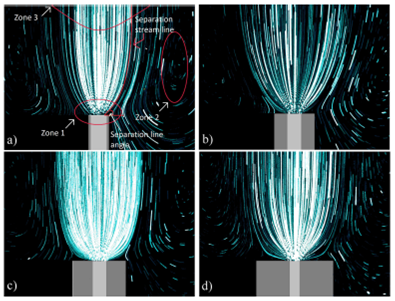FIGURE 3 Long exposure pictures of a submerged jet at Re = 0:11, t = 600 s, for: a)D in = 6:3 mm, e = R=4, b) D in = 6:3 mm, e = R=2, c) D in = 6:3 mm, e = R, and d) D in = 6:3 mm, e = 3RFIGURE 4 Plot of the decrease of the exit angle β when changing the thickness of the nozzle of the injector to Re = 0:11.

In Fig. 5, the distribution of the axial velocity component along the line of symmetry (maximum velocity) obtained from the experimental measurements is compared to the distribution produced by the numerical method developed for this study.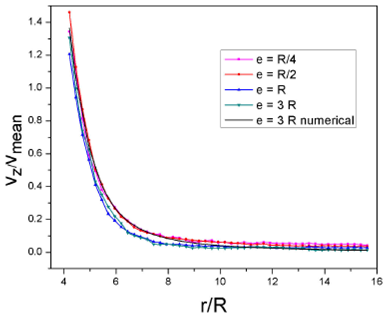FIGURE 5 Plot of the maximum speed drop vs. distance (r) at Re = 0:11.

In the numerical simulation, the change in the distribution of the axial velocity component that results from modifying the thickness of the tube is almost imperceptible, so the only distribution presented is the one corresponding to the thickest tube (e= 3R).

The axial velocity component distribution in the radial direction, at the positions R/2, R, 2R, 3R, 4R, and 5R, starting at the mouth of the tube, along the line of symmetry, were obtained, for a value of Re= 0.11, as shown in Figs. 5-12. In these plots, it is observed that by increasing the thickness of the wall of the tube, the width of the jet increases, causing the profile of the axial component of speed to contract in the axial direction and to elongate in the radial direction.FIGURE 6 Plot of the axial velocity component profile along the radial direction at the injector outlet (z 0) at Re = 0:11.FIGURE 7 Plot of the axial velocity component profile at z 0+0:5R distance from the nozzle outlet at Re = 0:11.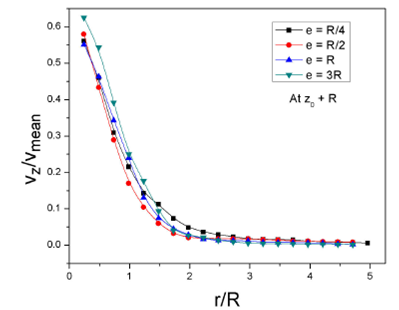FIGURE 8 Plot of the axial velocity component profile at z 0 + R distance from the injector outlet at Re = 0:11.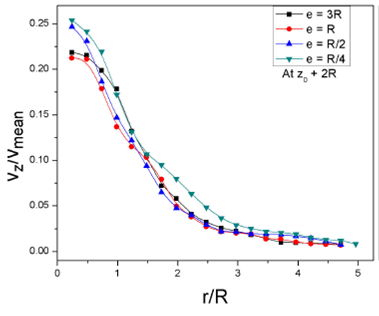FIGURE 9 Plot of the axial velocity component profile at z 0 +2R distance from the nozzle outlet at Re = 0:11.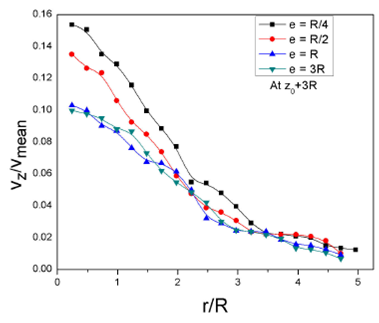FIGURE 10 Plot of the axial velocity component profile at z0+3R distance from the nozzle outlet at Re = 0:11.FIGURE 11 Plot of the axial velocity component profile at z0+4R distance from the injector outlet at Re = 0:11.FIGURE 12 Plot of the axial velocity component profile at z0+5R distance from the injector outlet at Re = 0:11.

Level curves of the dimensionless values of the velocity magnitude, the radial and axial components of the velocity, are placed side by side in Figs. 13 -15. Even though results were obtained for the four wall thicknesses, only the results for the case where e= 3R are presented. Similarly, in Figs. 16, the distribution of the axial velocity component along the radial direction at various positions along the symmetry line, as obtained experimentally, are compared with the numerically calculated values.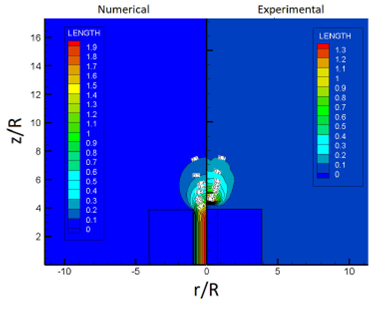FIGURE 13 Comparison of the velocity magnitude distribution for Re = 0:11; e = 3R, as obtained numerically and experimentally.FIGURE 14 Comparison of the radial velocity component distribution for Re = 0:11; e = 3R, as obtained numerically and experimentally.FIGURE 15 Comparison of the axial velocity component distribution for Re = 0:11; e = 3R, as obtained numerically and experimentally.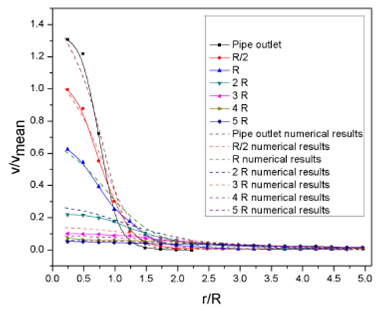FIGURE 16 Comparison of the profiles of the axial velocity component along the radial direction at various distances from the nozzle outlet, for Re = 0:11; e = 3R, as obtained numerically and experimentally.

To distinguish the main jet flow from the surrounding medium, it is considered that the jet, at all times, has the same volumetric flow rate as that occurring inside the tube.

The value of the stream function in the simulation is known since the numerical code that resolves the velocity is based on the vorticity-stream function formulation, which calculates the value of the stream function and subsequently derives the value of the velocity components.

To determine the value of the stream-function from the experimentally obtained values of the velocity components, a numerical code was developed, with a procedure similar to that described by Roache .

The value of the stream function corresponding to the separation streamline for the numerical simulation and the integration of the experimental results, is 0.5 and 0.48, respectively (see Fig. 17).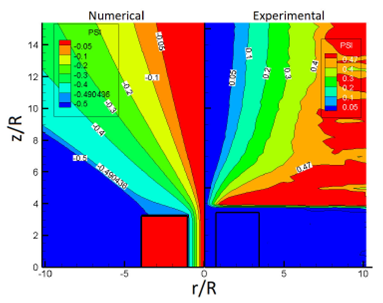FIGURE 17 Comparison of the stream-function (PSI) distribution for Re = 0:11; e = 3R, as obtained numerically and experimentally.

4.Discussion and conclusions

The structure of submerged jet flows originating from a circular tube and the actual effects that modifying the wall thickness of the tube has on such flows, for a given Reynolds number (Re= 0.11), were the studied experimentally and numerically. After comparing results, the following conclusions were reached:

• In practical jet flows, increasing the wall thickness of the injector increases the jet exit angle concerning the line of symmetry, also modifying the separation streamline between the main jet flow and the surrounding fluid.

• The change in the value of the exit angle is attributed to the occurrence of the no-slip condition at the edge of the injector.

• The change in the exit angle affects the width of the jet and the position of the recirculation cells.

• The velocity values and flow structure, obtained in this work by numerical and experimental methods are very similar between them.

It is considered that the change in the structure of the flow is translated into the change in the region of influence of the jet. It is expected that, at low Reynolds numbers, the fluid flow will be reversible, thus allowing to take samples from specific fluid regions.

Acknowledgments

M. Sanchez-Rosas and J. Casillas-Navarrete acknowledge CONACYT for a Ph D. studentship, A. Medina acknowledges partial support from Fondo SENER-Hidrocarburos Project 292334 CONACYT, for a stay at the UPM through the project: Fundamental models of the thermal methods of steam injection in EOR. He also acknowledges COTEBAL-IPN for a research annual leave. Finally, authors acknowledge Professor Amable Liñan from UPM for his illuminating comments and advices.

References

1. S. Vogel, Life in Moving Fluids: The Physical Biology of Flow, 2nd Ed. (Princeton University Press 1996), pp 78. [ Links ]

2. D. J. Doorly, D. J. Taylor, and R. C. Schroter, Respir. Physiol. Neurobiol. 163 (2008) 100. [ Links ]

4. H. B. Squire, Quart. Jour. Mech. Appl. Math. 4 (1951) 321. [ Links ]

5. V. I. Yatseyev, NACA TM 1349 (1953) 1. [ Links ]

6. A. Oberbeck, Ann. Phys. Chem. 49 (1877) 1. [ Links ]

7. A. Reynolds, J. Fluid Mech. 14 (1962) 552. doi:10.1017/S0022112062001433 [ Links ]

8. S. Abramovich, and A. Solan, J. Fluid Mech. 59 (1973) 791. doi:10.1017/S0022112073001886 [ Links ]

9. E. N. da C. Andrade and L. C. Tsien, Pro. Phys. Soc. 49 (1937) 381. [ Links ]

10. K. Mcnaughton, C. Sinclair, J. Fluid Mech. 25 (1966) 367.doi:10.1017/S0022112066001708 [ Links ]

11. W. Schneider, J. Fluid Mech. 108 (1981) 55. doi:10.1017/S0022112081001985 [ Links ]

12. E. Zauner, J. Fluid Mech. 154 (1985) 111. doi:10.1017/S0022112085001446 [ Links ]

13. W. Schneider, J. Fluid Mech. 154 (1985) 91. doi:10.1017/S0022112085001434 [ Links ]

14. V. V. Lemanov, Tech. Phys. Lett. 39 (2013) 421 . doi:10.1134/S1063785013050064 [ Links ]

15. An-Kuo Hsu, Jen-Yung Pu, Shuhn-Shyurng Hou, and Ta-Hui Lin, Int. J. Heat Mass Transf. 47 (2004) 1613. https://doi.org/10.1016/j.ijheatmasstransfer.2003.10.030 [ Links ]

16. D. S. Lee, K. D. Kihm and S. H. Chung, J. Fluids. Eng. 119 (1997) 716. doi:10.1115/1.2819304 [ Links ]

17. H. Kanda and S. Shimomukai, J. Complexity. 25 (2009) 253. [ Links ]

18. A. López-Villa, A. Medina, and F. J. Higuera, Phys. Fluids. 23 (2011) 102102. [ Links ]

19. Y. A. Çengel and J. M. Cimbala, Mecanica de fluidos fundamentos y aplicaciones, 1er Ed. (McgrawHill México 2006), pp. 892. [ Links ]

20. P. J. Roache, Fundamentals of Computational Fluid Dynamics, (Hermosa Albuquerque, New Mexico 1998), pp. 374. [ Links ]

Received: June 20, 2019; Accepted: August 13, 2019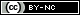This is an open-access article distributed under the terms of the Creative Commons Attribution License